﻿ 基于J函数的含油饱和度建模方法—以陆丰A油田为例 Modeling Method of Oil Saturation Based on J Function—Taking Lufeng A as an Example

Open Journal of Nature Science
Vol.05 No.05(2017), Article ID:22692,8 pages
10.12677/OJNS.2017.55060

Modeling Method of Oil Saturation Based on J Function

—Taking Lufeng A as an Example

Zong Dai1, Yidong Zhu1, Wei Li1, Zilong Ju1, Chen Xu2

1Institute of CNOOC Shenzhen Branch, Shenzhen Guangdong

2Essca Energy Science & Consulting Associate, Beijing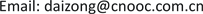Received: Oct. 27th, 2017; accepted: Nov. 9th, 2017; published: Nov. 16th, 2017ABSTRACT

With the development of water injection, the parameters of the reservoir change accordingly. Because of the influence of flooding, there is a significant difference between the oil saturation of logging interpretation and the original oil saturation of reservoir, and it cannot represent the original distribution of oil reservoir. According to the average capillary pressure curve, the original oil saturation of each type of reservoir is calculated by flow unit, and a model of oil saturation based on J function is established. The error of oil saturation in logging interpretation at different drilling periods is reduced. Therefore, this work is important for reserves recalculation and reservoir numerical simulation.

Keywords:Lufeng A Oil Fields, J Function, Saturation Model, Logging, Capillary Curve

—以陆丰A油田为例

1中海石油(中国)有限公司深圳分公司，广东 深圳

2阿什卡能源科学咨询服务联合公司，北京Copyright © 2017 by authors and Hans Publishers Inc.1. 引言

2. 基本原理

2.1. 利用J函数计算原始含油饱和度

${F}_{浮}=\Delta \rho gH$ (1)

${P}_{c}=\frac{2\sigma \mathrm{cos}\theta }{r}$ (2)

${P}_{c}=\Delta \rho gH$ (3)

${J}_{\left(Swn\right)}=\frac{{P}_{c}}{\sigma }\sqrt{\frac{K}{\phi }}$ (4)

${S}_{wn}=\frac{{S}_{w}-{S}_{wi}}{1-{S}_{wi}}$ (5)

${I}_{rq}=\sqrt{\frac{K}{\phi }}$ (6)

${P}_{c}\left(res\right)=\frac{{\sigma }_{res}\mathrm{cos}{\theta }_{res}}{{\sigma }_{lab}\mathrm{cos}{\theta }_{lab}}\ast {P}_{c}\left(lab\right)$ (7)

2.2. 基于J函数的含油饱和度建模

3. 实例运用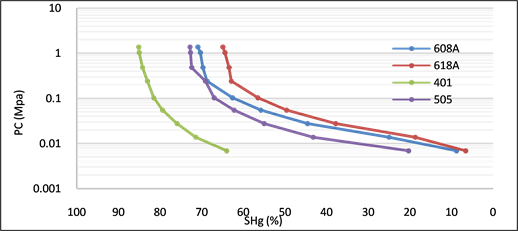Figure 1. Capillary pressure curve of the seventh sample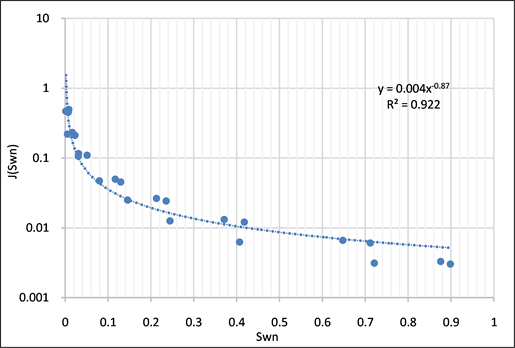Figure 2. J function fitting of the seventh sampleTable 1. Reservoir classification criteria and 11 types of J functions

1) 以相关反演结果为约束条件，采用续贯高斯方法模拟孔隙度分布。

2) 以孔隙度模型为约束条件，采用续贯高斯方法模拟渗透率分布。

3) 在孔隙度、渗透率模型的基础上，根据上述11类储层分类标准，计算储层分类模型。

4) 结合流体界面深度认识及界面张力、润湿角等参数，分不同储层类型完成基于J函数的饱和度建模。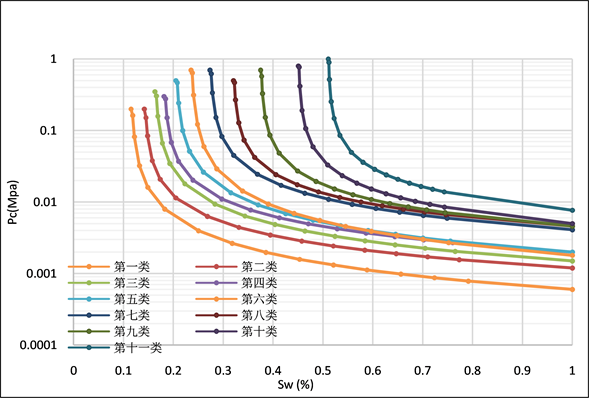Figure 3. Mean capillary pressure curve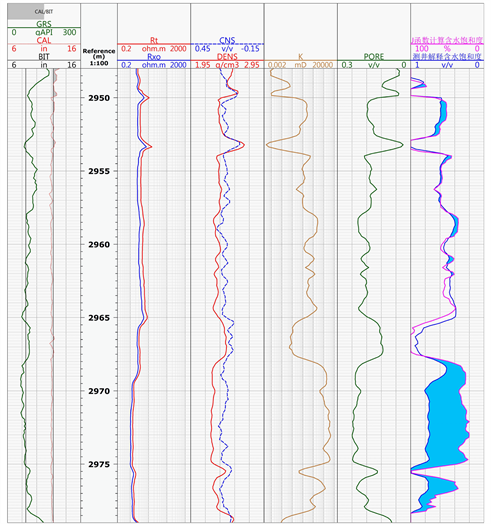Figure 4. Water saturation comparison between calculation values using drilling J function and logging interpretation value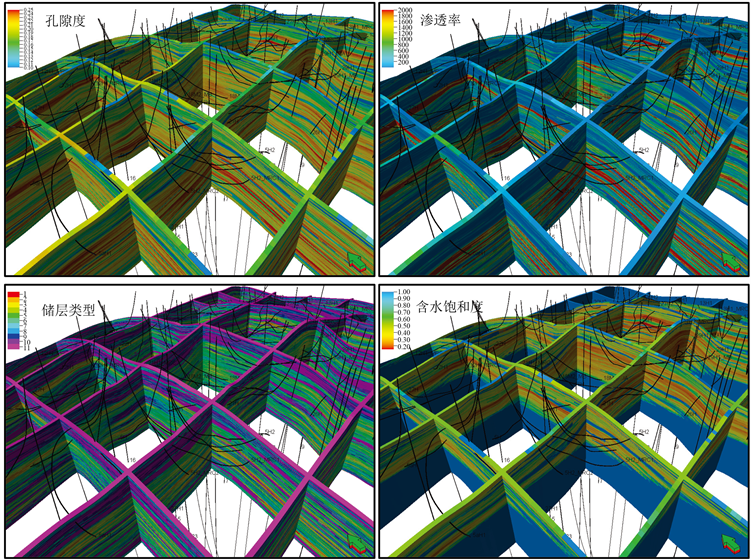Figure 5. Geological model of main parameters in the Lufeng A block

4. 主要结论

1) 通过J函数计算原始含油饱和度的方法，计算的结果只与储层孔隙结构、油水密度、油柱高度有关，与电阻率无关，从而解决了水淹层测井解释含油饱和度不能代表原始含油饱和度的问题；利用储层质量系数分类的方法，充分考虑了储层的孔隙结构差异，准确地计算各类储层的原始水饱和度，在非均质性强的储层中得到了很好的运用。

2) 基于J函数方法建立的含油饱和度模型，与不同岩石类型相渗一致，能够体现不同岩石类型毛细管力所引起的流体过渡带，与传统插值方法所建立的含油饱和度模型相比，能够更加真实地反映油气藏原始含油饱和度分布。

Modeling Method of Oil Saturation Based on J Function—Taking Lufeng A as an Example[J]. 自然科学, 2017, 05(05): 437-444. http://dx.doi.org/10.12677/OJNS.2017.55060

1. 1. 程超, 蔡华, 廖恒杰, 等. 海上油气田J函数约束饱和度地质建模方法[J]. 石油地质与工程, 2016, 30(1): 103-105.

2. 2. 陈康, 赵新军. 确定储层含油饱和度的测井——毛管资料综合法[J]. 江汉石油学院学报, 1995, 17(2): 51-55.

3. 3. 赵国欣, 朱家俊, 关丽. 用毛管压力资料求取原始含油饱和度的方法[J]. 中国石油大学学报(自然科学版), 2008, 32(4): 38-41.

4. 4. 唐衔, 侯加根, 许凡, 等. 利用压汞曲线求取油藏原始含油饱和度方法的研究——以克拉玛依油田为例[J]. 吐哈油气, 2008, 13(4): 366-399.

5. 5. 邵才瑞, 张鹏飞, 张福明, 等. 用J函数提高致密砂岩气层饱和度测井评价精度[J]. 中国石油大学学报(自然科学版), 2015, 40(4): 57-65.

6. 6. 廖敬, 彭彩珍, 吕文均, 等. 毛管压力曲线平均化及J函数处理[J]. 特种油气藏, 2008, 15(6): 73-75.

7. 7. 卢艳. 水淹层原始含水饱和度评价方法研究[J]. 国外测井技术, 2015(1): 16-19.

8. 8. Leverett, M.C. (1941) Capil-lary Behavior in Porous Solids. Transactions of the AIME, 142, 151-169.

9. 9. 司马立强, 李清, 杨毅, 等. 用J函数法求取碳酸盐岩储层饱和度方法探讨[J]. 岩性油气藏, 2014, 26(6): 106-110.

10. 10. 胡勇, 于兴河, 陈恭洋, 等. 平均毛管压力函数分类及其在流体饱和度计算中的应用[J]. 石油勘探与开发, 2012, 39(6): 733-738.

11. 11. 章成广, 秦瑞宝. 用毛管压力曲线解释原始含水饱和度[J]. 江汉石油大学学报, 1999, 21(4): 8-10.

12. 12. 管耀. 利用毛管压力资料求原始含油饱和度方法探讨[J]. 勘探地球物理进展, 2009, 32(5): 365-369.